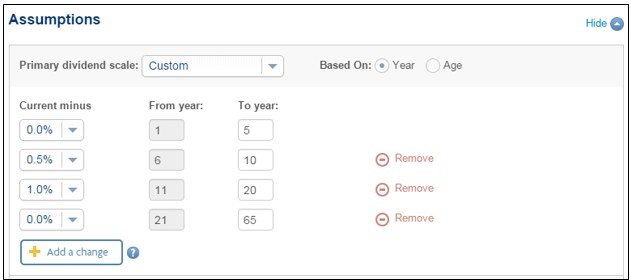# Custom dividend scales

The Situation:

You're illustrating participating insurance and you want to show projected values based on something other than a flat projected dividend scale.

How to illustrate a custom dividend scale

• In the Assumptions section, from the Primary dividend scale drop down list, select Custom.
• Depending on how you want to customize the illustration, for Based on, select either Year or Age.
• The illustration initially shows a single assumption but you can Add a change up to four times (for a total of five assumption layers). Each assumption layer can include any of the five available options which range from the current dividend scale down to current less 2%.
• Select the To year or age for each layer. The From year is automatically adjusted to be the year after the previous layer.

The following example shows a scenario that starts with the current dividend scale, reduces over time to Current less 1%, and then comes back up to the Current scale at year 21.Here are some things to be aware of when you customize the dividend scale.

The illustration always includes 3 scenarios:

The illustration always includes calculations based on 3 different dividend scales.

If you do not customize the dividend scale, the system calculates values based on:

• the Current scale,
• the Current scale less 1%, and
• the Current scale less 2%.

If you customize the dividend scale, the custom scale replaces the Current less 1% scenario. So in this situation the system calculates values based on:

• the Current scale,